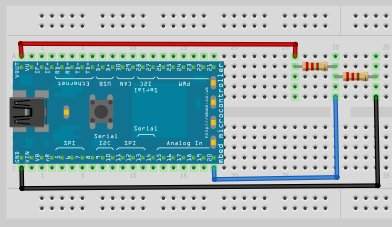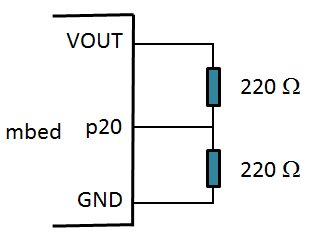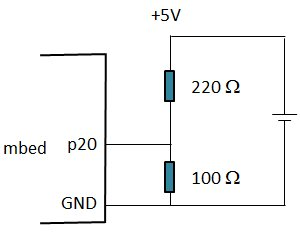### Example 1

1. Measure voltage using voltage divider rule. You can make use of different combination of resistors.```#include "mbed.h"

DigitalOut myled(LED1);

int main() {
while (1){
if(ain > 0.3) {
myled = 1;
} else {
myled = 0;
}
}
}
```

### Example 2If you need to measure voltage from other power source, you need to ensure that

1. The maximum voltage to mbed must be less than +3.3 V but larger than 0 V.
2. The ground pin from mbed must be tied to reference point of measured point.
3. mbed has a noise issue with analog input. You may want to use average to smooth out the data.
```/*
* The program try to test the phenomenon as mentioned in
*
*  a) Unused ADC pins are either tied to ground, or declared as DigitalOut
*  b) Quality of signal source, including low noise design techniques such
*     as filtering.
*  c) Reduce debugging communication via USB
*/

#include "mbed.h"

#define   SSIZE 100

BusOut    unused(p15,p16,p17,p18,p19);  // Make unused analog pin to DigitalOut

float     value[SSIZE];
float     max;
float     min;
double    itg;

void printNow()
{
time_t    s;
char      buffer;

s = time(NULL);
strftime(buffer, sizeof(buffer), "%Y-%m-%d %H:%M:%S", localtime(&s));
printf("%s\n", buffer);
}

void printSecNow()
{
time_t    s;
s = time(NULL);
printf("%d\n", s);
}

int main()
{
set_time(1323180910);

while (1)
{
//printNow();
printf("\n-----------------\n");
printSecNow();
max = itg = 0.0;
min = 3.3;
// Sample before printing to avoid debug communucation
for (int i=0; i<SSIZE; i++)
{
value[i] = ain;
if (value[i] > max) max = value[i];
if (value[i] < min) min = value[i];
itg += value[i];
}
printSecNow();

// Now print out
for (int i=0; i<SSIZE; i++)
{
printf("%0.3f ", value[i]*3.3);
}
printf("\n");
printf("max=%0.3f, min=%0.3f, avg=%0.3f\n",
max*3.3, min*3.3, 3.3*itg/SSIZE);
wait(10);
}
}
```